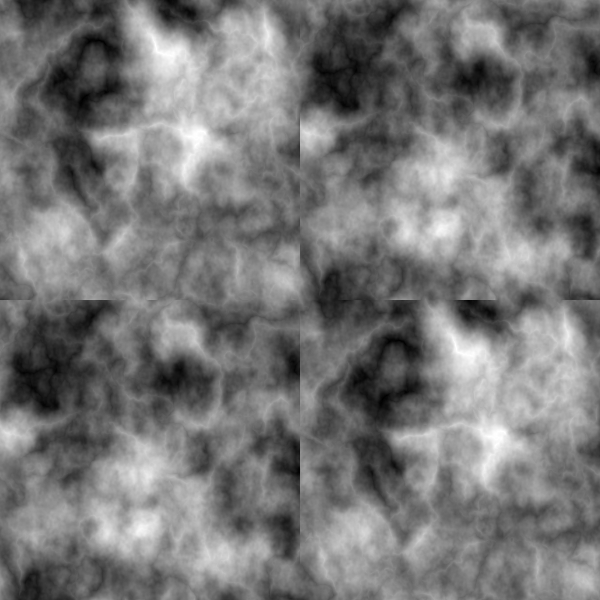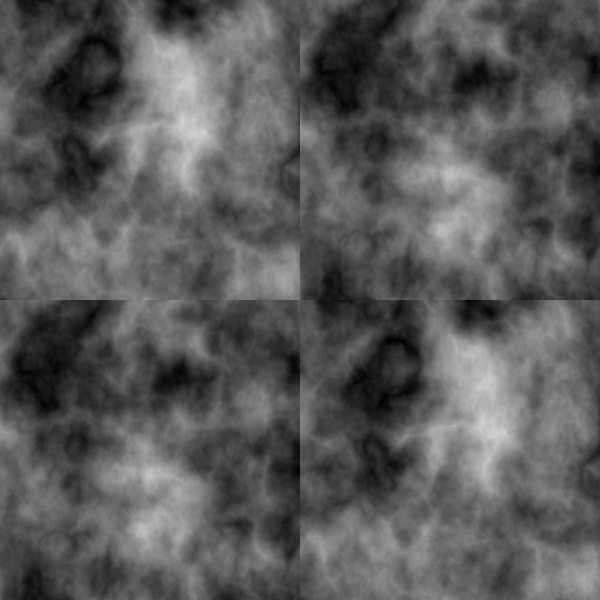## Recommended Posts

Exactly like that...assuming you did it with code, and not by making a circular ellipse and then resizing it.

Sorry, Reshaped it. :?

But it works just as well, for the moment. Would be cool if it's a tool.

##### Share on other sites
Exactly like that...assuming you did it with code, and not by making a circular ellipse and then resizing it.

Sorry, Reshaped it. :?

But it works just as well, for the moment. Would be cool if it's a tool.

##### Share on other sites

Hey is the plugin supposed to be located in a special folder in the effect list? I just cant seem to find it althought I'm pretty sure I put it in the right folder..##### Share on other sites

Hey is the plugin supposed to be located in a special folder in the effect list? I just cant seem to find it althought I'm pretty sure I put it in the right folder..##### Share on other sites
I do not understand well what you are saying!

But it makes me think about the Fragment plugin (from Ed Harvey)

http://paintdotnet.12.forumer.com/viewtopic.php?t=2969

Here's the best I can explain it...

...

sorry for rambling...So, who knows about plugins and ellipses?

I took the "Texture Helper" from Boltbait, and instead moving the pixel, I make an average with Source+Destination.

http://paintdotnet.12.forumer.com/viewtopic.php?t=2614

Test the code with CodeLab:

```void Render(Surface dst, Surface src, Rectangle rect)
{
PdnRegion selectionRegion = EnvironmentParameters.GetSelection(src.Bounds);
Rectangle selection = this.EnvironmentParameters.GetSelection(src.Bounds).GetBoundsInt();
int CenterX = (int)(((selection.Right - selection.Left) / 2) + selection.Left);
int CenterY = (int)(((selection.Bottom - selection.Top) / 2) + selection.Top);
ColorBgra PixD,Pix1,Pix2;
int srcX = 0, srcY = 0;

for(int y = rect.Top; y < rect.Bottom; y++)
{
for (int x = rect.Left; x < rect.Right; x++)
{
srcX = x;
srcY = y;

if (x < CenterX) srcX += CenterX;
else srcX -= CenterX;

if (y < CenterY) srcY += CenterY;
else srcY -= CenterY;

Pix1 = src[srcX,srcY];
Pix2 = src[x,y];
PixD = src[x,y];
PixD.R = (byte)((float)(Pix1.R + Pix2.R + 0.5f)/2.0f);
PixD.G = (byte)((float)(Pix1.G + Pix2.G + 0.5f)/2.0f);
PixD.B = (byte)((float)(Pix1.B + Pix2.B + 0.5f)/2.0f);
PixD.A = (byte)((float)(Pix1.A + Pix2.A + 0.5f)/2.0f);
dst[x,y] = PixD;
}
}
}```

##### Share on other sites
I do not understand well what you are saying!

But it makes me think about the Fragment plugin (from Ed Harvey)

http://paintdotnet.12.forumer.com/viewtopic.php?t=2969

Here's the best I can explain it...

...

sorry for rambling...So, who knows about plugins and ellipses?

I took the "Texture Helper" from Boltbait, and instead moving the pixel, I make an average with Source+Destination.

http://paintdotnet.12.forumer.com/viewtopic.php?t=2614

Test the code with CodeLab:

```void Render(Surface dst, Surface src, Rectangle rect)
{
PdnRegion selectionRegion = EnvironmentParameters.GetSelection(src.Bounds);
Rectangle selection = this.EnvironmentParameters.GetSelection(src.Bounds).GetBoundsInt();
int CenterX = (int)(((selection.Right - selection.Left) / 2) + selection.Left);
int CenterY = (int)(((selection.Bottom - selection.Top) / 2) + selection.Top);
ColorBgra PixD,Pix1,Pix2;
int srcX = 0, srcY = 0;

for(int y = rect.Top; y < rect.Bottom; y++)
{
for (int x = rect.Left; x < rect.Right; x++)
{
srcX = x;
srcY = y;

if (x < CenterX) srcX += CenterX;
else srcX -= CenterX;

if (y < CenterY) srcY += CenterY;
else srcY -= CenterY;

Pix1 = src[srcX,srcY];
Pix2 = src[x,y];
PixD = src[x,y];
PixD.R = (byte)((float)(Pix1.R + Pix2.R + 0.5f)/2.0f);
PixD.G = (byte)((float)(Pix1.G + Pix2.G + 0.5f)/2.0f);
PixD.B = (byte)((float)(Pix1.B + Pix2.B + 0.5f)/2.0f);
PixD.A = (byte)((float)(Pix1.A + Pix2.A + 0.5f)/2.0f);
dst[x,y] = PixD;
}
}
}```

##### Share on other sites

i like the plugin.

##### Share on other sites

i like the plugin.

##### Share on other sites
...I make an average with Source+Destination...

Is this "average" like The Lighten blending mode?

##### Share on other sites
...I make an average with Source+Destination...

Is this "average" like The Lighten blending mode?

##### Share on other sites
...I make an average with Source+Destination...

Is this "average" like The Lighten blending mode?

I don't know how The Lighten blending mode works!

Pixel Destination = (Pixel1 + Pixel2) /2

PixD.R = (Pix1.R + Pix2.R + 0.5)/2

PixD.G = (Pix1.G + Pix2.G + 0.5)/2

PixD.B = (Pix1.B + Pix2.B + 0.5)/2

PixD.A = (Pix1.A + Pix2.A + 0.5)/2

##### Share on other sites
...I make an average with Source+Destination...

Is this "average" like The Lighten blending mode?

I don't know how The Lighten blending mode works!

Pixel Destination = (Pixel1 + Pixel2) /2

PixD.R = (Pix1.R + Pix2.R + 0.5)/2

PixD.G = (Pix1.G + Pix2.G + 0.5)/2

PixD.B = (Pix1.B + Pix2.B + 0.5)/2

PixD.A = (Pix1.A + Pix2.A + 0.5)/2

##### Share on other sites
Test the code with CodeLab:

```void Render(Surface dst, Surface src, Rectangle rect)
...
dst[x,y] = PixD;
}
}
}```

I get a compile error in codelab that PixD is an unassigned local variable. (line 41, which is the "dst[x,y] = PixD" one).

I'd very much like to understand what this code is doing, but I don't know enough about the colorBGRa type to understand why it would be unassigned after 4 lines of code that appear to assign values to its color channel properties.

##### Share on other sites
Test the code with CodeLab:

```void Render(Surface dst, Surface src, Rectangle rect)
...
dst[x,y] = PixD;
}
}
}```

I get a compile error in codelab that PixD is an unassigned local variable. (line 41, which is the "dst[x,y] = PixD" one).

I'd very much like to understand what this code is doing, but I don't know enough about the colorBGRa type to understand why it would be unassigned after 4 lines of code that appear to assign values to its color channel properties.

##### Share on other sites
Test the code with CodeLab:

```void Render(Surface dst, Surface src, Rectangle rect)
...
dst[x,y] = PixD;
}
}
}```

I get a compile error in codelab that PixD is an unassigned local variable. (line 41, which is the "dst[x,y] = PixD" one).

I'd very much like to understand what this code is doing, but I don't know enough about the colorBGRa type to understand why it would be unassigned after 4 lines of code that appear to assign values to its color channel properties.

Fixed! See above...

##### Share on other sites
Test the code with CodeLab:

```void Render(Surface dst, Surface src, Rectangle rect)
...
dst[x,y] = PixD;
}
}
}```

I get a compile error in codelab that PixD is an unassigned local variable. (line 41, which is the "dst[x,y] = PixD" one).

I'd very much like to understand what this code is doing, but I don't know enough about the colorBGRa type to understand why it would be unassigned after 4 lines of code that appear to assign values to its color channel properties.

Fixed! See above...

##### Share on other sites
...I make an average with Source+Destination...

Is this "average" like The Lighten blending mode?

I don't know how The Lighten blending mode works!

Pixel Destination = (Pixel1 + Pixel2) /2

PixD.R = (Pix1.R + Pix2.R + 0.5)/2

PixD.G = (Pix1.G + Pix2.G + 0.5)/2

PixD.B = (Pix1.B + Pix2.B + 0.5)/2

PixD.A = (Pix1.A + Pix2.A + 0.5)/2

They are different!

Using Lighten Blending Mode:##### Share on other sites
...I make an average with Source+Destination...

Is this "average" like The Lighten blending mode?

I don't know how The Lighten blending mode works!

Pixel Destination = (Pixel1 + Pixel2) /2

PixD.R = (Pix1.R + Pix2.R + 0.5)/2

PixD.G = (Pix1.G + Pix2.G + 0.5)/2

PixD.B = (Pix1.B + Pix2.B + 0.5)/2

PixD.A = (Pix1.A + Pix2.A + 0.5)/2

They are different!

Using Lighten Blending Mode:##### Share on other sites

Well, so why not a chooser for blend mode!

As an example :

```int Amount1=2;	//[1,3]1=Lighten,2=Average,3=Darken
void Render(Surface dst, Surface src, Rectangle rect)
{
PdnRegion selectionRegion = EnvironmentParameters.GetSelection(src.Bounds);
Rectangle selection = this.EnvironmentParameters.GetSelection(src.Bounds).GetBoundsInt();
int CenterX = (int)(((selection.Right - selection.Left) / 2) + selection.Left);
int CenterY = (int)(((selection.Bottom - selection.Top) / 2) + selection.Top);
ColorBgra PixD,Pix1,Pix2;
int srcX = 0, srcY = 0;
for(int y = rect.Top; y < rect.Bottom; y++)
{
for (int x = rect.Left; x < rect.Right; x++)
{
srcX = x;
srcY = y;

if (x < CenterX) srcX += CenterX;
else srcX -= CenterX;

if (y < CenterY) srcY += CenterY;
else srcY -= CenterY;

Pix1 = src[srcX,srcY];
Pix2 = src[x,y];
PixD = src[x,y];
if (Amount1==1)
{
PixD.R = (byte)(Math.Max(Pix1.R,Pix2.R));
PixD.G = (byte)(Math.Max(Pix1.G,Pix2.G));
PixD.B = (byte)(Math.Max(Pix1.B,Pix2.);
PixD.A = (byte)(Math.Max(Pix1.A,Pix2.A));
}
else if (Amount1==2)
{
PixD.R = (byte)((float)(Pix1.R + Pix2.R) / 2.0f + 0.5f);
PixD.G = (byte)((float)(Pix1.G + Pix2.G) / 2.0f + 0.5f);
PixD.B = (byte)((float)(Pix1.B + Pix2. / 2.0f + 0.5f);
PixD.A = (byte)((float)(Pix1.A + Pix2.A) / 2.0f + 0.5f);
}
else if (Amount1==3)
{
PixD.R = (byte)(Math.Min(Pix1.R,Pix2.R));
PixD.G = (byte)(Math.Min(Pix1.G,Pix2.G));
PixD.B = (byte)(Math.Min(Pix1.B,Pix2.);
PixD.A = (byte)(Math.Min(Pix1.A,Pix2.A));
}
dst[x,y] = PixD;
}
}
}```

##### Share on other sites

Well, so why not a chooser for blend mode!

As an example :

```int Amount1=2;	//[1,3]1=Lighten,2=Average,3=Darken
void Render(Surface dst, Surface src, Rectangle rect)
{
PdnRegion selectionRegion = EnvironmentParameters.GetSelection(src.Bounds);
Rectangle selection = this.EnvironmentParameters.GetSelection(src.Bounds).GetBoundsInt();
int CenterX = (int)(((selection.Right - selection.Left) / 2) + selection.Left);
int CenterY = (int)(((selection.Bottom - selection.Top) / 2) + selection.Top);
ColorBgra PixD,Pix1,Pix2;
int srcX = 0, srcY = 0;
for(int y = rect.Top; y < rect.Bottom; y++)
{
for (int x = rect.Left; x < rect.Right; x++)
{
srcX = x;
srcY = y;

if (x < CenterX) srcX += CenterX;
else srcX -= CenterX;

if (y < CenterY) srcY += CenterY;
else srcY -= CenterY;

Pix1 = src[srcX,srcY];
Pix2 = src[x,y];
PixD = src[x,y];
if (Amount1==1)
{
PixD.R = (byte)(Math.Max(Pix1.R,Pix2.R));
PixD.G = (byte)(Math.Max(Pix1.G,Pix2.G));
PixD.B = (byte)(Math.Max(Pix1.B,Pix2.);
PixD.A = (byte)(Math.Max(Pix1.A,Pix2.A));
}
else if (Amount1==2)
{
PixD.R = (byte)((float)(Pix1.R + Pix2.R) / 2.0f + 0.5f);
PixD.G = (byte)((float)(Pix1.G + Pix2.G) / 2.0f + 0.5f);
PixD.B = (byte)((float)(Pix1.B + Pix2. / 2.0f + 0.5f);
PixD.A = (byte)((float)(Pix1.A + Pix2.A) / 2.0f + 0.5f);
}
else if (Amount1==3)
{
PixD.R = (byte)(Math.Min(Pix1.R,Pix2.R));
PixD.G = (byte)(Math.Min(Pix1.G,Pix2.G));
PixD.B = (byte)(Math.Min(Pix1.B,Pix2.);
PixD.A = (byte)(Math.Min(Pix1.A,Pix2.A));
}
dst[x,y] = PixD;
}
}
}```

##### Share on other sites
For me it's in Distort

AHA!

Thx a lot

## Join the conversation

You can post now and register later. If you have an account, sign in now to post with your account.